Disclosure: This post may contain affiliate links, meaning when you click the links and make a purchase, we receive a commission.

# Compound interest excel formula with regular deposits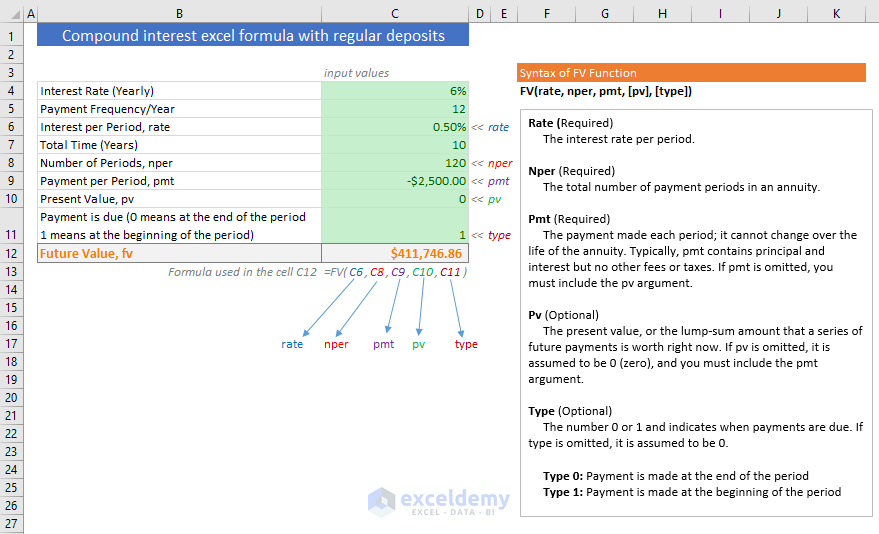In this tutorial, I will explain how to calculate compound interest using Excel formula with regular and irregular deposits. We shall also discuss how to calculate future values of an investment on the basis of daily, monthly and yearly compounding interest rate.

Compounding interest rate concept is the center point of the investment world. It moves the stock market, the bond market or simply the world. Understanding compounding interest rate can change your behavior with money and savings.

For individuals who did not study finance or accounting or business studies, the concepts might seem a little bit complex. But if you read this article with attention, your misconceptions will be removed, your understandings will be clear.

## Compound interest excel formula with regular deposits (Using Excel FV Function)

Say, you’re going to run a savings scheme with one of your trusted banks. And you want to know what will be your total amount after a certain period of time (years).

In this case, you will have to use Excel’s FV function.

Excel’s FV function returns the future value of an investment based on periodic, constant payments and a constant interest rate.

Syntax of Excel FV function: FV(rate, nper, pmt, [pv], [type])

Here,

• rate (required) is the interest rate per period. If the yearly interest rate is 6% and payments are made every month per period rate will be 6%/12 = 0.5%
• nper (required) is the total number of periods. If the total number of years is 10 years but payments are made every month, the total number of periods is 10 x 12 = 120
• pmt (required) is the amount of payment made each period
• pv (optional) is the present value of the investment
• type (optional) payments can be made at the end of the period (omitted or value 0), or payments can be done at the start of the period (type is 1).

Example 1

• Annual percentage rate, APR = 6%
• Payment Frequency/Year = 12
• Interest per Period, rate = 6%/12 = 0.5%
• Total Time (Years) = 10
• Number of Periods, nper = 10 x 12 = 120
• Payment per Period, pmt = -2500 (-ve as this is cash outflows)
• Present Value, pv = 0
• Payment made at the beginning of the Period: 1

This is the result when we use FV function on the above values.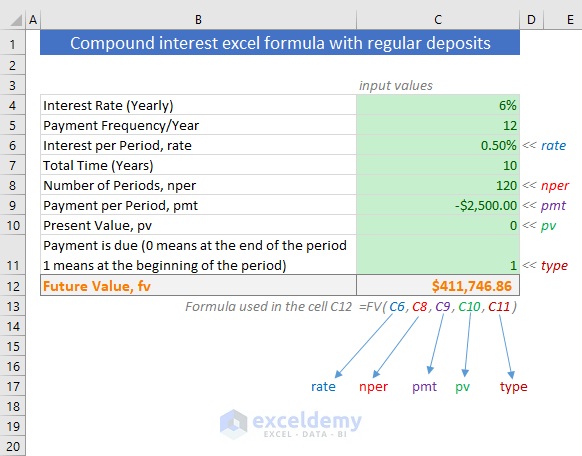So, with a monthly payment of 2500\$, and annual interest rate 6%, after making 120 periods, you can expect your return is \$409698.37

Now you can change the input values as per your need.

Example 2

Now say, you want to make some initial investment, then you want to make your regular deposits.

• Annual percentage rate, APR = 6%
• Payment Frequency/Year = 12
• Interest per Period, rate = 6%/12 = 0.5%
• Total Time (Years) = 10
• Number of Periods, nper = 10 x 12 = 120
• Payment per Period, pmt = -2500 (-ve as this is cash outflows)
• Present Value, pv = -50000 (it is also -ve as it is cash outflow)
• Payment made at the beginning of the Period: 1

This is what we get as return after 10 years of monthly payments.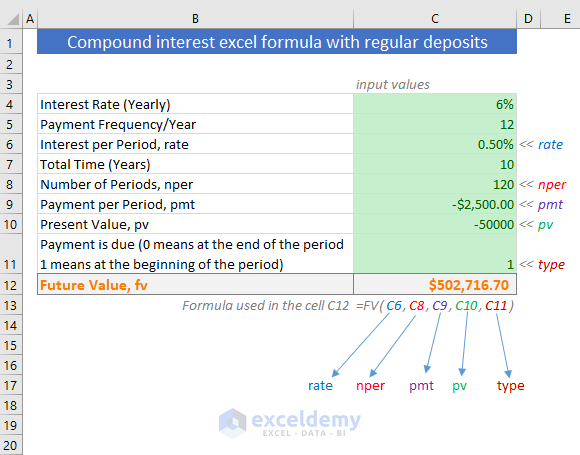Now use this template to calculate for daily, weekly, monthly, or any number of payment frequencies.

## Calculating compound interest with regular deposits (Using no function)

In the above section, we have seen how to calculate the compound interest rate with regular deposits using Excel’s FV function.

But without using FV function, we can also calculate the compound interest with regular deposits, even with irregular deposits.

In the following image, you see, we have calculated the ‘Amount at the end of the period’ in both ways:

• Using Excel FV function
• And using regular Excel formulas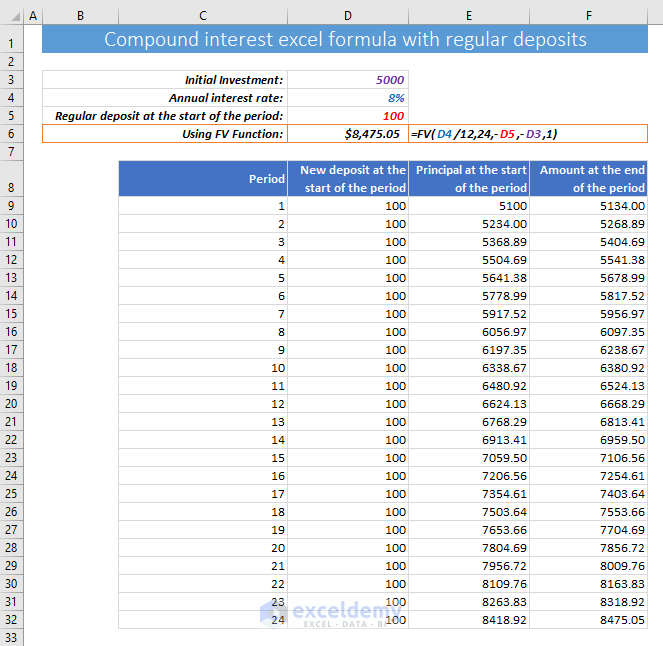Let me explain in step by step how I made this Excel template:

• For simplicity, I have taken only 24 months or periods (under the Period column). Add more periods under this column if necessary and apply the formulas from the above row.
• In the cell D9 (under the column “New deposit at the start of the period”), I have used this formula, D9=\$D\$5. And then applied this formula for other cells in the column.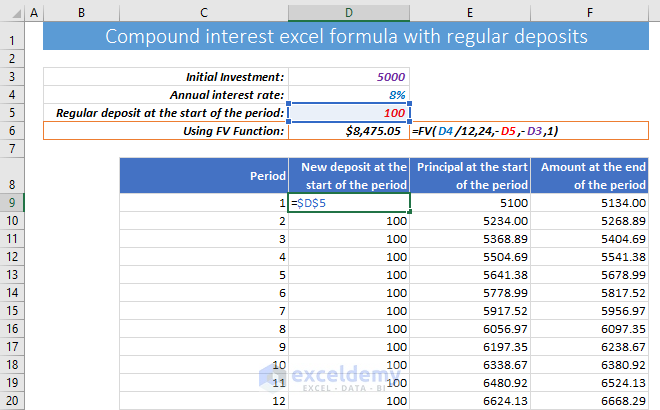• In the cell E9 (under the column “Principal at the start of the period”), I have used this formula, E9=D9+D3. This formula is used just for once. This is just to add the initial investment to the formula.

Note: this is not a practice to start a deposit scheme with an initial investment. I have just shown it for the explanation. In most of the cases, the initial investment will be zero.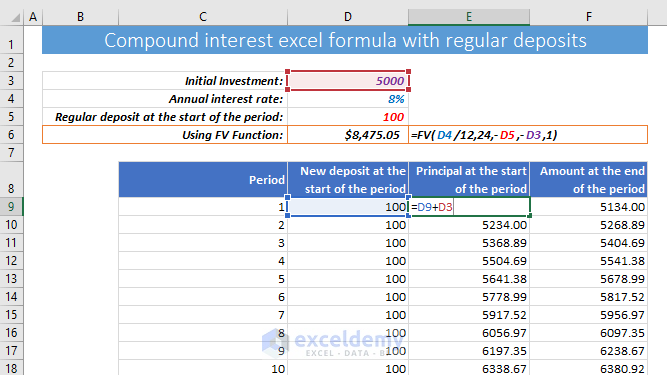• In the cell F9 (under the column Amount at the end of the period), I have used this formula, F9=E9+E9*(\$D\$4/12)

This formula will add the Principal at the start of the period (E9) to the interest earned (E9*(\$D\$4/12)) for the period. We are dividing the yearly interest rate \$D\$4 by 12 as the regular deposit is made monthly. Copy the formula and apply it to the cells below.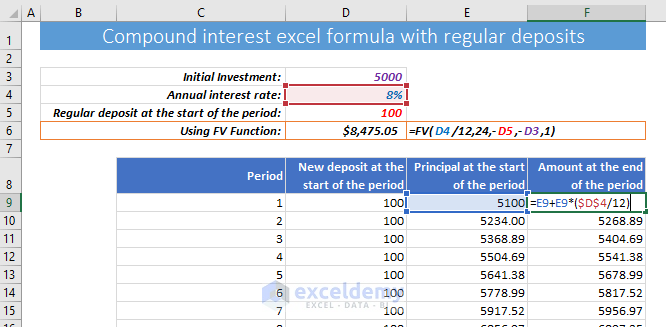• In the cell E10 (under the column Principal at the start of the period), I have used this formula, E10=F9+D10. This formula will add the new deposit to the amount at the end of the previous period. And then I have copied down this formula for other cells in the column.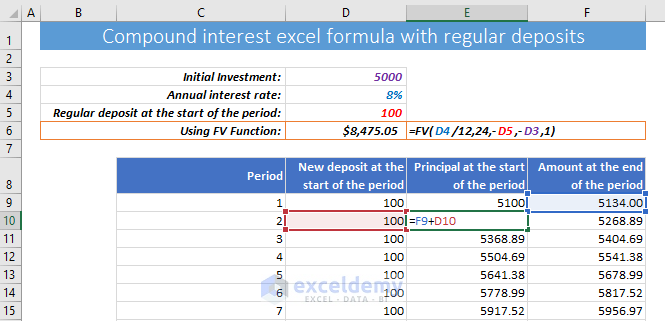## Calculate compound interest with irregular deposits

I can extend the previous template to calculate compound interest with irregular deposits. Just use your irregular deposits manually in the “New deposit at the start of the period” column like the image below.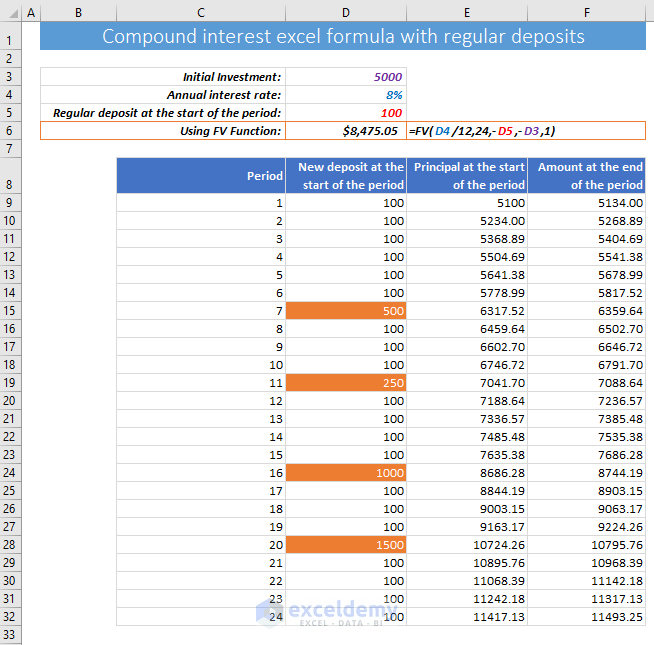## Compounding interest definition & building compound interest formula

Suppose, you have some investable money of amount \$10,000.

You go to a bank and the bank said their savings rate is 6% per year.

You deposited the money with the bank for the next 3 years as you felt safe with the bank and the interest rate is competitive.

Annual interest rate is: 6%

### After 1 year

After 1 year, you will receive interest of amount: \$10,000 x 6% = \$10,000 x (6/100) = \$600

So, after 1 year, your principal + interest will be:

= \$10,000 + \$600

= \$10,000 + \$10,000 x 6%; [replacing \$600 with \$10,000 x 6%]

= \$10,000 (1+6%)

If you withdraw this interest (\$600), then your principal at the beginning of the 2nd year will be \$10,000.

But if you don’t withdraw the interest, your principal at the beginning of the 2nd year will be: \$10,000 + \$600 = \$10,600

And this is where compounding starts.

When you don’t withdraw the interest, the interest is added to your principal. The principal and earned interest work as your new principal for the next year.

Your next year’s interest is calculated based on this new principal.

Eventually, the yearly return from investments in the coming years gets bigger.

### After 2 years

At the beginning of year 2, your new principal is: \$10,600

At the end of the year 2, you will receive interest (on the basis of new principal) of amount: \$10,600 x 6% = \$636

So, after 2 years, your principal + interest will be:

= \$10,600 + \$636

Let’s make the compound interest rate formula from the above expression:

= \$10,000(1+6%) + \$10,600 x 6%; [replacing \$10,600 with \$10,000(1+6%) and \$636 with \$10,600 x 6%]

= \$10,000(1+6%) + \$10,000(1+6%) x 6%; [again replacing \$10,600 with \$10,000(1+6%)]

= \$10,000(1+6%)(1+6%)

= \$10,000 x (1+6%)^2

So, we can make a generalized compound interest formula to calculate principal + interest:

=p(1+r)^n

Where,

• p is the principal invested at the beginning of the annuity,
• r is the yearly interest rate (APR)
• And n is the number of years.

So, your principal + interest at the end of the year 2 will be: \$10600 + \$636 = \$11,236

We can also reach this same amount using the above formula:

=p(1+r)^n

=\$10,000 x (1+6%)^2

= \$10,000 (1+0.06)^2

= \$10,000 (1.06)^2

=\$10,000 x 1.1236

= \$11,236

### After 3 years

The new principal at the start of year 3 is: \$11,236

But we don’t need this to calculate the principal + interest at the end of year 3. We can use the formula directly.

After 3 years, your principal + interest will be:

= \$10,000 x (1+6%)^3

= \$11,910.16

## More robust compound interest formula

This is the more accurate and all-in-one formula to calculate the compound interest rate.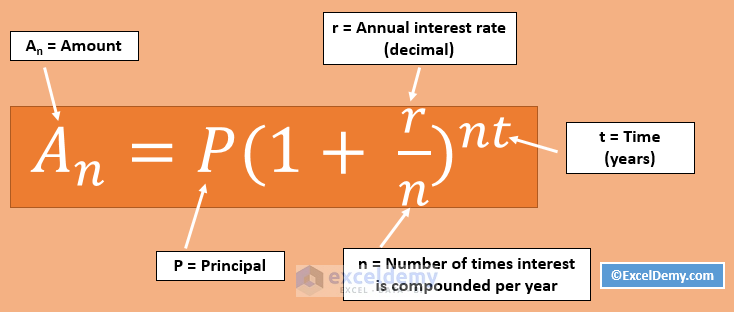A = P (1 + r/n) (nt)

Where,

• A = Total amount after nt periods
• P = The amount invested at the beginning. It cannot be withdrawn or changed in the investment period.
• r = Annual Percentage Rate (APR)
• n = Number of times interest is compounded per year
• t = Total time in years

We shall see several uses of the above formula.

Using this formula, we can calculate future values of investment on the basis of daily, monthly, and yearly compounding interest rate.

## Futures values of an investment using compound interest formula (for daily, weekly, monthly, and quarterly)

Using the following compound interest formula, we can calculate futures values on investment for any compounding frequency.

A = P (1 + r/n) (nt)

Check out the image below. I have shown 4 variations of the above formula.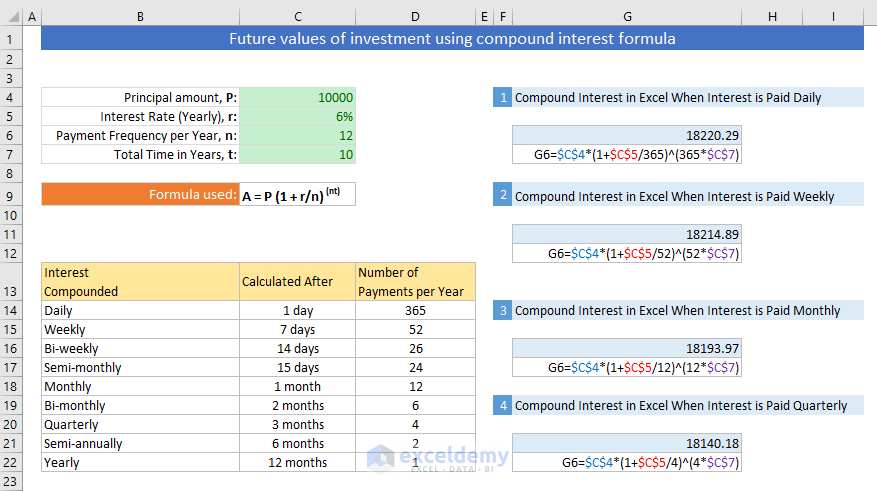You see that for the same investment of amount \$10,000, we get the following results:

• For daily compounding: \$18220.29
• For weekly compounding: \$18214.89
• For Monthly compounding: \$18193.97
• And for Quarterly compounding: \$18140.18

So, if the number of compounding per year is higher, the return is also higher.

## Power of Compounding. Why saving is important in your life?

Let me show you the power of compounding in the investment world or with your savings.

Suppose, you want to be a millionaire and that is in sleeping mode 😊

Warren Buffet (the living legend of the investment world) advises you to invest in a low-cost index fund, for example, Vanguard 500 Index Investor. And historically this fund has returned 8.33% annual return for the last 15 years (including the fall of 2008).

So, how much do you need to be a millionaire, and how much time?

Here is an assumption: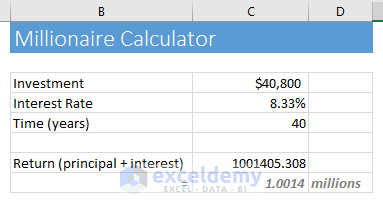Suppose, your age is now 25 and you have saved 40,000\$ (I am assuming, you’re a good saver). And your plan is to retire after 40 years. Invest the fund with the VFINX index fund and forget about it.

After 40 years, check out your return. More or less, you will get around 1 million dollars from this tiny investment.

Let me show you now the return after 40 years when your yearly return is a few basis points higher than 8.33%.

Say, you are an active trader and you can manage a 13% return a year. It is quite possible.

What will be your return? See the result.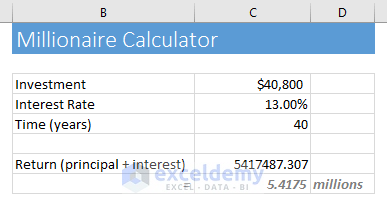With an annual compound return of 13%, your \$40,800 will be 5.42 million after 40 years. Amazing, right?

Warren Buffet, the greatest investor of our time, compounded his money at the rate of 21% every year, for the last 50 years of investment periods.

Just see the gain if you can do so: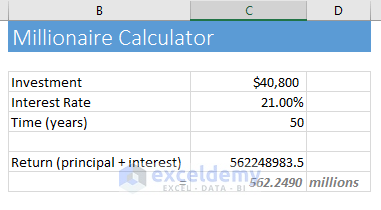In a period of 50 years, with an annual return of 21%, your tiny amount of \$40,800 will make you half a billion-dollar.

This is the power of compounding.